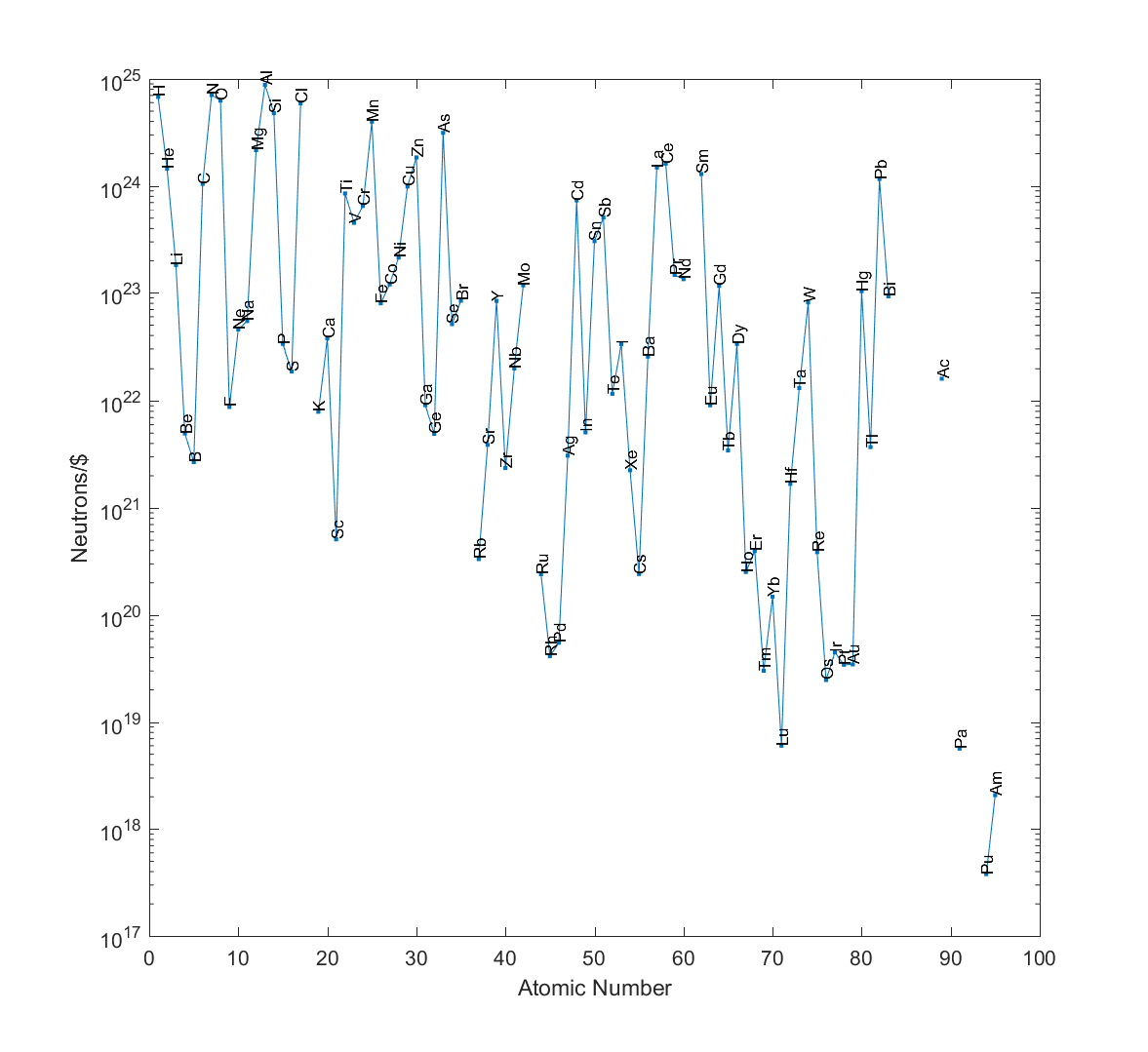# How much for that neutron in the window?

Zach Weinersmith asked:

That is a great question. I once came up with the answer “50 tons of neutrons are needed” to a serious problem (you don’t want to know). How cheaply could you get that?

Figuring out roughly how many neutrons there are per kilogram of pure elements is pretty easy. Get their standard atomic weights,$A$, and subtract the atomic number$Z$ since that is the number of protons:$N=A-Z$. Now we know how many neutrons there are per atom on average (standard atomic weights include the different isotope weights, weighted by their abundance).

[ Since nucleons (protons and neutrons) are about 1830 times heavier than electrons, we can ignore the electrons for an error on order of 0.05%. There is also a binding energy error, since some of the total atomic mass is because of binding energy between nucleons, which is 0.94% or less. These errors are nothing compared to price uncertainties.]

We know that one nucleon weighs about$u=1.660539040\cdot 10^{-27}$ kg, so the number of nucleons per kilogram is$N_{\mathrm{nucl}} \approx 1/(Au)$ and the number of neutrons per kilo is$N_n \approx N_{\mathrm{nucl}}(N/A)$. This ranges from$7.5\cdot 10^{25}$ for helium down to$1.2\cdot 10^{24}$ for Oganesson. Hydrogen just has$4.7\cdot 10^{24}$ neutrons per kilogram, despite having$5.97\cdot 10^{26}$ nucleons per kilogram – there isn’t that much deuterium and tritium around to contribute neutrons.

Now, the price of elements is badly defined. I can get a kilogram of coal much cheaper than a kilogram of diamond, and ultra-pure elements are very expensive even if the everyday element is cheap. Plus, prices vary. And it is hard to buy plutonium on the open market. Ignoring all that and taking the numbers from Wikipedia (and ignoring the that some values look odd, and some are for compounds, and that the prices are unadjusted for inflation, and that they are lacking for many elements…) we can actually calculate the number of neutrons per dollar:Neutrons per dollar if one buys one kilogram of the element.

And the winner is… aluminium! You can get$8.8\cdot 10^{24}$ neutrons per dollar from aluminium.

In second place, nitrogen ($7.1\cdot 10^{24}$) and in third, hydrogen ($6.8\cdot 10^{24}$)! Hydrogen may be very neutron-poor, but since it is rather cheap and you get lots of nucleons per kilo, this balances the lack.

Given that these prices are dodgy, I would expect an uncertainty on the order of a magnitude (at least). So the true winner, given the cheapest actual source of the element, might be hard to find without excruciating price comparisons. But we can be fairly certain it is going to be something with an atomic number less than 25. Uranium is unlikely to be a cheap neutron source in this sense (and just look at poor plutonium!)

So, given that aluminium is 51.8% neutrons by weight I need 96.5 tons. The current aluminium price is $1,650.00 per ton, so I would have to pay$159,225 for the neutrons in my doomsday weapon – I mean, totally innocuous thought experiment!